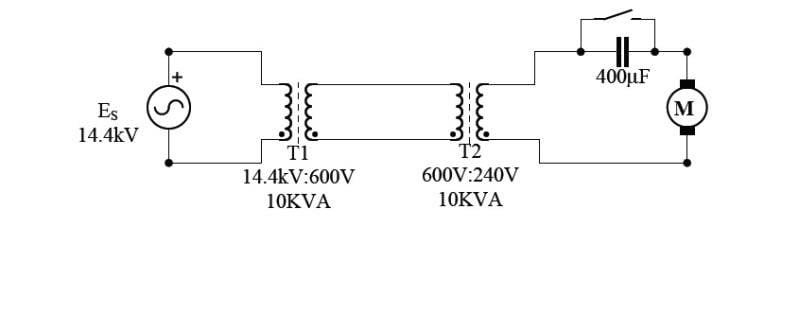# Circuit analysis (with transformers and motor)

• Engineering

## Homework StatementConsider the motor arrangement shown, Assume ideal transformers and lossless transmission lines. The start capacitor shown in series with the motor is only used to start the motor, and is then shorted out of the circuit. The motor is loaded such that it delivers 5hp, and has a power factor of 0.6 lagging, and the motors efficiency is 90%.

A)Find the impedence of the normally running motor (ie. capacitor shorted).
$$P_{out}=5hp=3730W, P_{in}=3730W/0.9=4144W, F_P=0.6$$
$$Z=V^2/S$$
$$S=\sqrt{Q^2+P_{in}^2}=\sqrt{(tan(cos^{-1}(F_P))P_{in})^2+P_{in}^2}= \sqrt{(tan(cos^{-1}(0.6))4144W)^2+(4144W)^2}=6907VAR$$
and
$$Z=(240V)^2/6907VAR=8.34Ω$$

B) What impedence is seen by the source Es? What is the magnitude of the source current?
Firstly, I considered the motor segment to be "secondary".
$$Z_S=n^2Z_P,\rightarrow{Z}_P=Z_S(e_2/e_p)^2=(8.34Ω)(240V/14.4(10)^3V)^2=2.32mΩ$$